# Minimum over principal ideal of Euclidean norm is a smaller multiplicatively monotone Euclidean norm

## Statement

Suppose$R$ is a commutative unital ring and$N$ is a Euclidean norm on$R$ -- in particular,$R$ is a Eulidean ring. We can define a new Euclidean norm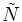$\tilde{N}$ on$R$ as follows (for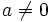$a \ne 0$):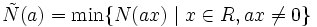$\tilde{N}(a) = \min \{ N(ax) \mid x \in R, ax \ne 0 \}$.

In other words, it is the minimum of the norms of nonzero elements in the principal ideal generated by$a$.$\tilde{N}$ is a multiplicatively monotone norm and further,$\tilde{N}(a) \le N(a)$ for any$a \ne 0$. Thus,$\tilde{N}$ is a smaller multiplicatively monotone Euclidean norm on$R$.

## Proof

Given: A ring$R$ with a Euclidean norm$N$. Define, for$a \ne 0$,$\tilde{N}(a) = \min \{ N(ax) \mid x \in R, ax \ne 0 \}$.

To prove:$\tilde{N}$ is Euclidean,$\tilde{N}(ab) \ge \max \{ \tilde{N}(a), \tilde{N}(b) \}$ for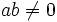$ab \ne 0$, and$\tilde{N}(a) \le N(a)$ for$a \ne 0$.

Proof:

1. Proof that$\tilde{N}(a) \le N(a)$ for all$a \ne 0$: This is direct since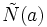$\tilde{N}(a)$ is the minimum over a collection of numbers that includes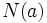$N(a)$.
2. Proof that$\tilde{N}(ab) \ge \max \{ \tilde{N}(a), \tilde{N}(b)\}$ for$ab \ne 0$: This follows from the fact that the set of nonzero multiples of$ab$ is contained in the set of nonzero multiples of$a$, as well as in the set of nonzero multiples of$b$.
3. Proof that$\tilde{N}$ is Euclidean: Suppose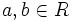$a,b \in R$ with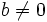$b \ne 0$. Then,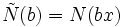$\tilde{N}(b) = N(bx)$ for some$x \in R$. Euclidean division of$a$ by$bx$ with respect to the original norm$N$ yields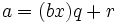$a = (bx)q + r$ where$r = 0$ or$N(r) < N(bx)$. In particular,$r = 0$ or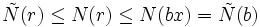$\tilde{N}(r) \le N(r) \le N(bx) = \tilde{N}(b)$. Thus, we have$a = b(xq) + r$ where$r = 0$ or$\tilde{N}(r) < \tilde{N}(b)$.